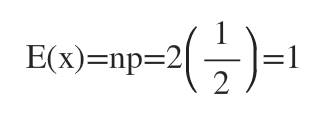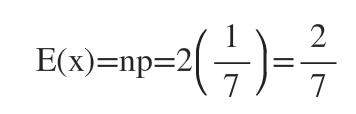# Rework problem 15 from section 4.3 of your text, involving the expected number of heads when flipping one of two coins. One coin is fair and one is weighted so that Pr[H]=1/7. You randomly select one of the two coins, and flip it 2 times, noting the result of each flip.What is the expected number of heads?

Question
11 views

Rework problem 15 from section 4.3 of your text, involving the expected number of heads when flipping one of two coins. One coin is fair and one is weighted so that Pr[H]=1/7. You randomly select one of the two coins, and flip it 2 times, noting the result of each flip.

What is the expected number of heads?

check_circle

Step 1

Let, X= the number of heads

X follows a binomial distribution with parameters n and p.

For the fair coin n= 2 and p=1/2

So the expected number of heads on the fair coin is:For the weighted coin n=2 and p=1/7

So, the expected number of heads on the weighted coin is:...

### Want to see the full answer?

See Solution

#### Want to see this answer and more?

Solutions are written by subject experts who are available 24/7. Questions are typically answered within 1 hour.*

See Solution
*Response times may vary by subject and question.
Tagged in